16. Each of the nine small squares in this grid can be coloured completely black or completely white.

What is the largest number of squares that can be coloured black so that the design created has rotational symmetry of order 2, but no lines of symmetry?

A:
B:
C:
D:
E:

17. In a group of 48 children, the ratio of boys to girls is 3 : 5.

How many boys must join the group to make the ratio of boys to girls 5 : 3?

A:
B:
C:
D:
E:

18. In the addition sum shown, each letter represents a different non-zero digit.

What digit does X represent?

A:
B:
C:
D:
E:

19. Three boxes under my stairs contain apples or pears or both. Each box contains the same number of pieces of fruit. The first box contains all twelve of the apples and one-ninth of the pears.

How many pieces of fruit are there in each box?

A:
B:
C:
D:
E:

20. A cyclic quadrilateral has all four vertices on the circumference of a circle.

Brahmagupta (598–670AD) gave the following formula for the area, A, of a cyclic quadrilateral whose edges have lengths a, b, c, d:

where s is half of the perimeter of the quadrilateral.

What is the area of the cyclic quadrilateral with sides of length 4cm, 5cm, 7cm and 10cm?

21. The diagram shows a pentagon drawn on a square grid. All vertices of the pentagon and triangle are grid points.

What fraction of the area of the pentagon is shaded?

A: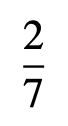B: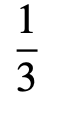C: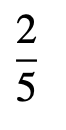D: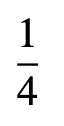E: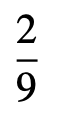22. Four copies of the triangle shown are joined together, without gaps or overlaps, to make a parallelogram.

What is the largest possible perimeter of the parallelogram?

23. The diagram shows the first few squares of a ‘spiral’ sequence of squares. All but the first three squares have been numbered.

After the first six squares, the sequence is continued by placing the next square alongside three existing squares – the largest existing square and two others.

The three smallest squares have sides of length 1.

What is the side length of the 12th square?

A:B:C:D:E:24. Part of a wall is to be decorated with a row of four square tiles. Three different colours of tiles are available and there are at least two tiles of each colour available.

Tiles of all three colours must be used.

In how many ways can the row of four tiles be chosen?

A:
B:
C:
D:
E:

25. Beatrix places dominoes on a 5 × 5 board, either horizontally or vertically, so that each domino covers two small squares.

She stops when she cannot place another domino, as in the example shown in the diagram.

When Beatrix stops, what is the largest possible number of squares that may be still uncovered?

A:B:C:D:E:Back to the top